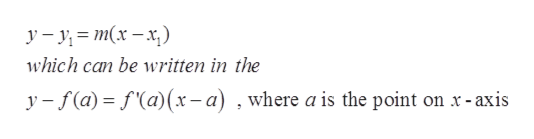# A function f has f(5) = 20 and f ′(5) = 2, and f ′′(x) < 0 for x ≥ 5. Which of the following values for f(7) is possible __________________________________ ?. Hint draw a picture. Find the equation of the tangent line and use the tangent line to help discover which value is possible. The supplement to No 6 Day Test will help with this question.     a.26 b.24 c.22 d.10 e.none of these

Question
39 views

A function f has f(5) = 20 and f ′(5) = 2, and f ′′(x) < 0 for x ≥ 5. Which of the following values for f(7) is possible __________________________________ ?.

Hint draw a picture. Find the equation of the tangent line and use the tangent line to help discover which value is possible. The supplement to No 6 Day Test will help with this question.

 a. 26 b. 24 c. 22 d. 10 e. none of these
check_circle

Step 1

Given,

Step 2

The tangent line is given byhelp_outlineImage Transcriptionclosey m(x-x) which can be written in the y-f(a) fa)(x-a) , where a is the point on x-axis fullscreen
Step 3

Here, a = 5

Plugging value of a...

### Want to see the full answer?

See Solution

#### Want to see this answer and more?

Solutions are written by subject experts who are available 24/7. Questions are typically answered within 1 hour.*

See Solution
*Response times may vary by subject and question.
Tagged in

### Derivative